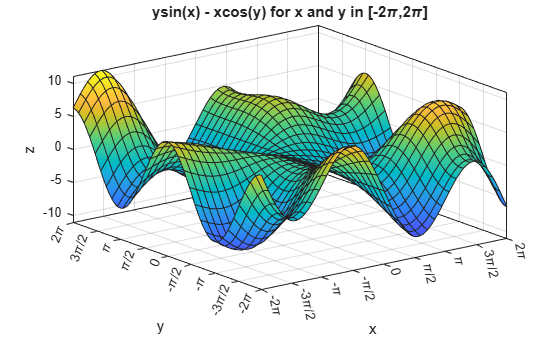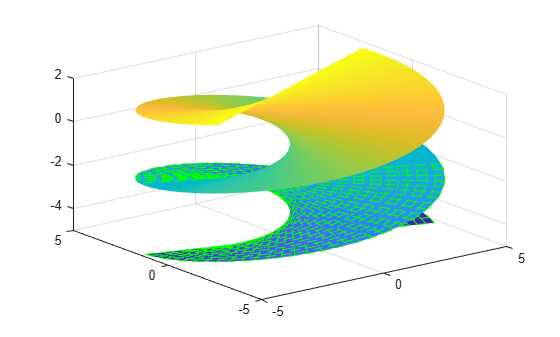fsurf

Plot 3-D surface

Description

example

fsurf(f) creates a surface plot of the function z = f(x,y) over the default interval [-5 5] for x and y.

fsurf(f,xyinterval) plots over the specified interval. To use the same interval for both x and y, specify xyinterval as a two-element vector of the form [min max]. To use different intervals, specify a four-element vector of the form [xmin xmax ymin ymax].

example

fsurf(funx,funy,funz) plots the parametric surface defined by x = funx(u,v), y = funy(u,v), z = funz(u,v) over the default interval [-5 5] for u and v.

fsurf(funx,funy,funz,uvinterval) plots over the specified interval. To use the same interval for both u and v, specify uvinterval as a two-element vector of the form [min max]. To use different intervals, specify a four-element vector of the form [umin umax vmin vmax].

fsurf(___,LineSpec) sets the line style, marker symbol, and surface color. For example, '-r' specifies red lines. Use this option after any of the previous input argument combinations.

example

fsurf(___,Name,Value) specifies surface properties using one or more name-value pair arguments. Use this option after any of the input argument combinations in the previous syntaxes.

fsurf(ax,___) plots into the axes specified by ax instead of the current axes (gca).

example

fs = fsurf(___) returns a FunctionSurface object or ParameterizedFunctionSurface object, depending on the inputs. Use fs to query and modify properties of a specific surface. For a list of properties, see FunctionSurface Properties or ParameterizedFunctionSurface Properties.

Examples

collapse all

Plot the expression $\mathrm{sin}\left(x\right)+\mathrm{cos}\left(y\right)$ over the default interval $-5 and $-5.

fsurf(@(x,y) sin(x)+cos(y))Plot the piecewise expression

$\begin{array}{cc}erf\left(x\right)+\mathrm{cos}\left(y\right)& -5

over $-5

Specify the plotting interval as the second input argument of fsurf. When you plot multiple surfaces over different intervals in the same axes, the axis limits adjust to include all the data.

f1 = @(x,y) erf(x)+cos(y);
fsurf(f1,[-5 0 -5 5])
hold on
f2 = @(x,y) sin(x)+cos(y);
fsurf(f2,[0 5 -5 5])
hold offPlot the parameterized surface

$\begin{array}{c}x=r\mathrm{cos}\left(u\right)\mathrm{sin}\left(v\right)\\ y=r\mathrm{sin}\left(u\right)\mathrm{sin}\left(v\right)\\ z=r\mathrm{cos}\left(v\right)\\ where\phantom{\rule{1em}{0ex}}r=2+\mathrm{sin}\left(7u+5v\right)\end{array}$

for $0 and $0. Add light to the surface using camlight.

r = @(u,v) 2 + sin(7.*u + 5.*v);
funx = @(u,v) r(u,v).*cos(u).*sin(v);
funy = @(u,v) r(u,v).*sin(u).*sin(v);
funz = @(u,v) r(u,v).*cos(v);
fsurf(funx,funy,funz,[0 2*pi 0 pi])
camlightFor $x$ and $y$ from $-2\pi$ to $2\pi$, plot the 3-D surface $y\mathrm{sin}\left(x\right)-x\mathrm{cos}\left(y\right)$. Add a title and axis labels and display the axes outline.

fsurf(@(x,y) y.*sin(x)-x.*cos(y),[-2*pi 2*pi])
title('ysin(x) - xcos(y) for x and y in [-2\pi,2\pi]')
xlabel('x');
ylabel('y');
zlabel('z');
box onSet the x-axis tick values and associated labels using the XTickLabel and XTick properties of axes object. Access the axes object using gca. Similarly, set the y-axis tick values and associated labels.

ax = gca;
ax.XTick = -2*pi:pi/2:2*pi;
ax.XTickLabel = {'-2\pi','-3\pi/2','-\pi','-\pi/2','0',...
'\pi/2','\pi','3\pi/2','2\pi'};

ax.YTick = -2*pi:pi/2:2*pi;
ax.YTickLabel = {'-2\pi','-3\pi/2','-\pi','-\pi/2','0',...
'\pi/2','\pi','3\pi/2','2\pi'};Plot the parametric surface $x=u\mathrm{sin}\left(v\right)$, $y=-u\mathrm{cos}\left(v\right)$, $z=v$ with different line styles for different values of $v$. For $-5, use a dashed green line for the surface edges. For $-2, turn off the edges by setting the EdgeColor property to 'none'.

funx = @(u,v) u.*sin(v);
funy = @(u,v) -u.*cos(v);
funz = @(u,v) v;

fsurf(funx,funy,funz,[-5 5 -5 -2],'--','EdgeColor','g')
hold on
fsurf(funx,funy,funz,[-5 5 -2 2],'EdgeColor','none')
hold offPlot the parametric surface

$\begin{array}{c}x={e}^{-|u|/10}\mathrm{sin}\left(5|v|\right)\\ y={e}^{-|u|/10}\mathrm{cos}\left(5|v|\right)\\ z=u.\end{array}$

Assign the parameterized function surface object to a variable.

x = @(u,v) exp(-abs(u)/10).*sin(5*abs(v));
y = @(u,v) exp(-abs(u)/10).*cos(5*abs(v));
z = @(u,v) u;
fs = fsurf(x,y,z)fs =
ParameterizedFunctionSurface with properties:

XFunction: @(u,v)exp(-abs(u)/10).*sin(5*abs(v))
YFunction: @(u,v)exp(-abs(u)/10).*cos(5*abs(v))
ZFunction: @(u,v)u
EdgeColor: [0 0 0]
LineStyle: '-'
FaceColor: 'interp'

Show all properties

Change the plotting interval for u to [-30 30] by setting the URange property of object. Add transparency to the surface by setting the FaceAlpha property to a value between 0 (transparent) and 1 (opaque).

fs.URange = [-30 30];
fs.FaceAlpha = .5;Show contours below a surface plot by setting the 'ShowContours' option to 'on'.

f = @(x,y) 3*(1-x).^2.*exp(-(x.^2)-(y+1).^2)...
- 10*(x/5 - x.^3 - y.^5).*exp(-x.^2-y.^2)...
- 1/3*exp(-(x+1).^2 - y.^2);
fsurf(f,[-3 3],'ShowContours','on')Control the resolution of a surface plot using the 'MeshDensity' option. Increasing 'MeshDensity' can make smoother, more accurate plots while decreasing it can increase plotting speed.

Create two plots in a tiled chart layout. In the first plot, display the parametric surface $x=\mathrm{sin}\left(s\right)$, $y=\mathrm{cos}\left(s\right)$, $z=\left(t/10\right)\mathrm{sin}\left(1/s\right)$. The surface has a large gap. Fix this issue by increasing the 'MeshDensity' to 40 in the second plot. fsurf fills the gap, showing that by increasing 'MeshDensity' you increased the resolution.

tiledlayout(2,1)
nexttile
fsurf(@(s,t) sin(s), @(s,t) cos(s), @(s,t) t/10.*sin(1./s))
view(-172,25)
title('Default MeshDensity = 35')

nexttile
fsurf(@(s,t) sin(s), @(s,t) cos(s),...
@(s,t) t/10.*sin(1./s),'MeshDensity',40)

view(-172,25)
title('Increased MeshDensity = 40')Input Arguments

collapse all

3-D function to plot, specified as a function handle to a named or anonymous function.

Specify a function of the form z = f(x,y). The function must accept two matrix input arguments and return a matrix output argument of the same size. Use array operators instead of matrix operators for the best performance. For example, use .* (times) instead of * (mtimes).

Example: f = @(x,y) sin(x) + cos(y);

Plotting interval for x and y, specified in one of these forms:

• Vector of form [min max] — Use the interval [min max] for both x and y

• Vector of form [xmin xmax ymin ymax] — Use the interval [xmin xmax] for x and [ymin ymax] for y.

Parametric function for x coordinates, specified as a function handle to a named or anonymous function.

Specify a function of the form x = funx(u,v). The function must accept two matrix input arguments and return a matrix output argument of the same size. Use array operators instead of matrix operators for the best performance. For example, use .* (times) instead of * (mtimes).

Example: funx = @(u,v) u.*sin(v);

Parametric function for y coordinates, specified as a function handle to a named or anonymous function.

Specify a function of the form y = funy(u,v). The function must accept two matrix input arguments and return a matrix output argument of the same size. Use array operators instead of matrix operators for the best performance. For example, use .* (times) instead of * (mtimes).

Example: funy = @(t) @(u,v) -u.*cos(v);

Parametric function for z coordinates, specified as a function handle to a named or anonymous function.

Specify a function of the form z = funz(u,v). The function must accept two matrix input arguments and return a matrix output argument of the same size. Use array operators instead of matrix operators for the best performance. For example, use .* (times) instead of * (mtimes).

Example: funz = @(u,v) v;

Plotting interval for u and v, specified in one of these forms:

• Vector of form [min max] — Use the interval [min max] for both u and v

• Vector of form [umin umax vmin vmax] — Use the interval [umin umax] for u and [vmin vmax] for v.

Axes object. If you do not specify an axes object, then fsurf uses the current axes.

Line style, marker, and color, specified as a character vector or string containing symbols. The symbols can appear in any order. You do not need to specify all three characteristics (line style, marker, and color). For example, if you omit the line style and specify the marker, then the plot shows only the marker and no line.

Example: '--or' is a red dashed line with circle markers

Line StyleDescriptionResulting Line
'-'Solid line'--'Dashed line':'Dotted line'-.'Dash-dotted lineMarkerDescriptionResulting Marker
'o'Circle'+'Plus sign'*'Asterisk'.'Point'x'Cross'_'Horizontal line'|'Vertical line's'Square'd'Diamond'^'Upward-pointing triangle'v'Downward-pointing triangle'>'Right-pointing triangle'<'Left-pointing triangle'p'Pentagram'h'HexagramColor NameShort NameRGB TripletAppearance
'red''r'[1 0 0]'green''g'[0 1 0]'blue''b'[0 0 1]'cyan' 'c'[0 1 1]'magenta''m'[1 0 1]'yellow''y'[1 1 0]'black''k'[0 0 0]'white''w'[1 1 1]Name-Value Arguments

Specify optional comma-separated pairs of Name,Value arguments. Name is the argument name and Value is the corresponding value. Name must appear inside quotes. You can specify several name and value pair arguments in any order as Name1,Value1,...,NameN,ValueN.

Example: 'Marker','o','MarkerFaceColor','red'

The properties list here are only a subset. For a full list, see FunctionSurface Properties orParameterizedFunctionSurface Properties.

Number of evaluation points per direction, specified as a number. The default is 35. Because fsurf objects use adaptive evaluation, the actual number of evaluation points is greater.

Example: 100

Display contour plot under plot, specified as 'on' or 'off', or as numeric or logical 1 (true) or 0 (false). A value of 'on' is equivalent to true, and 'off' is equivalent to false. Thus, you can use the value of this property as a logical value. The value is stored as an on/off logical value of type matlab.lang.OnOffSwitchState.

Line color, specified as 'interp', an RGB triplet, a hexadecimal color code, a color name, or a short name. The default RGB triplet value of [0 0 0] corresponds to black. The 'interp' value colors the edges based on the ZData values.

For a custom color, specify an RGB triplet or a hexadecimal color code.

• An RGB triplet is a three-element row vector whose elements specify the intensities of the red, green, and blue components of the color. The intensities must be in the range [0,1]; for example, [0.4 0.6 0.7].

• A hexadecimal color code is a character vector or a string scalar that starts with a hash symbol (#) followed by three or six hexadecimal digits, which can range from 0 to F. The values are not case sensitive. Thus, the color codes '#FF8800', '#ff8800', '#F80', and '#f80' are equivalent.

Alternatively, you can specify some common colors by name. This table lists the named color options, the equivalent RGB triplets, and hexadecimal color codes.

Color NameShort NameRGB TripletHexadecimal Color CodeAppearance
'red''r'[1 0 0]'#FF0000''green''g'[0 1 0]'#00FF00''blue''b'[0 0 1]'#0000FF''cyan' 'c'[0 1 1]'#00FFFF''magenta''m'[1 0 1]'#FF00FF''yellow''y'[1 1 0]'#FFFF00''black''k'[0 0 0]'#000000''white''w'[1 1 1]'#FFFFFF''none'Not applicableNot applicableNot applicableNo color

Here are the RGB triplets and hexadecimal color codes for the default colors MATLAB® uses in many types of plots.

[0 0.4470 0.7410]'#0072BD'[0.8500 0.3250 0.0980]'#D95319'[0.9290 0.6940 0.1250]'#EDB120'[0.4940 0.1840 0.5560]'#7E2F8E'[0.4660 0.6740 0.1880]'#77AC30'[0.3010 0.7450 0.9330]'#4DBEEE'[0.6350 0.0780 0.1840]'#A2142F'Line style, specified as one of the options listed in this table.

Line StyleDescriptionResulting Line
'-'Solid line'--'Dashed line':'Dotted line'-.'Dash-dotted line'none'No lineNo line

Line width, specified as a positive value in points, where 1 point = 1/72 of an inch. If the line has markers, then the line width also affects the marker edges.

The line width cannot be thinner than the width of a pixel. If you set the line width to a value that is less than the width of a pixel on your system, the line displays as one pixel wide.

Marker symbol, specified as one of the values listed in this table. By default, the object does not display markers. Specifying a marker symbol adds markers at each data point or vertex.

MarkerDescriptionResulting Marker
'o'Circle'+'Plus sign'*'Asterisk'.'Point'x'Cross'_'Horizontal line'|'Vertical line's'Square'd'Diamond'^'Upward-pointing triangle'v'Downward-pointing triangle'>'Right-pointing triangle'<'Left-pointing triangle'p'Pentagram'h'Hexagram'none'No markersNot applicable

Marker size, specified as a positive value in points, where 1 point = 1/72 of an inch.

Output Arguments

collapse all

One or more FunctionSurface or ParameterizedFunctionSurface objects, returned as a scalar or a vector.

• If you use the fsurf(f) syntax or a variation of this syntax, then fsurf returns FunctionSurface objects.

• If you use the fsurf(funx,funy,funz) syntax or a variation of this syntax, then fsurf returns ParameterizedFunctionSurface objects.

You can use these objects to query and modify properties of a specific surface. For a list of properties, see FunctionSurface Properties and ParameterizedFunctionSurface Properties.